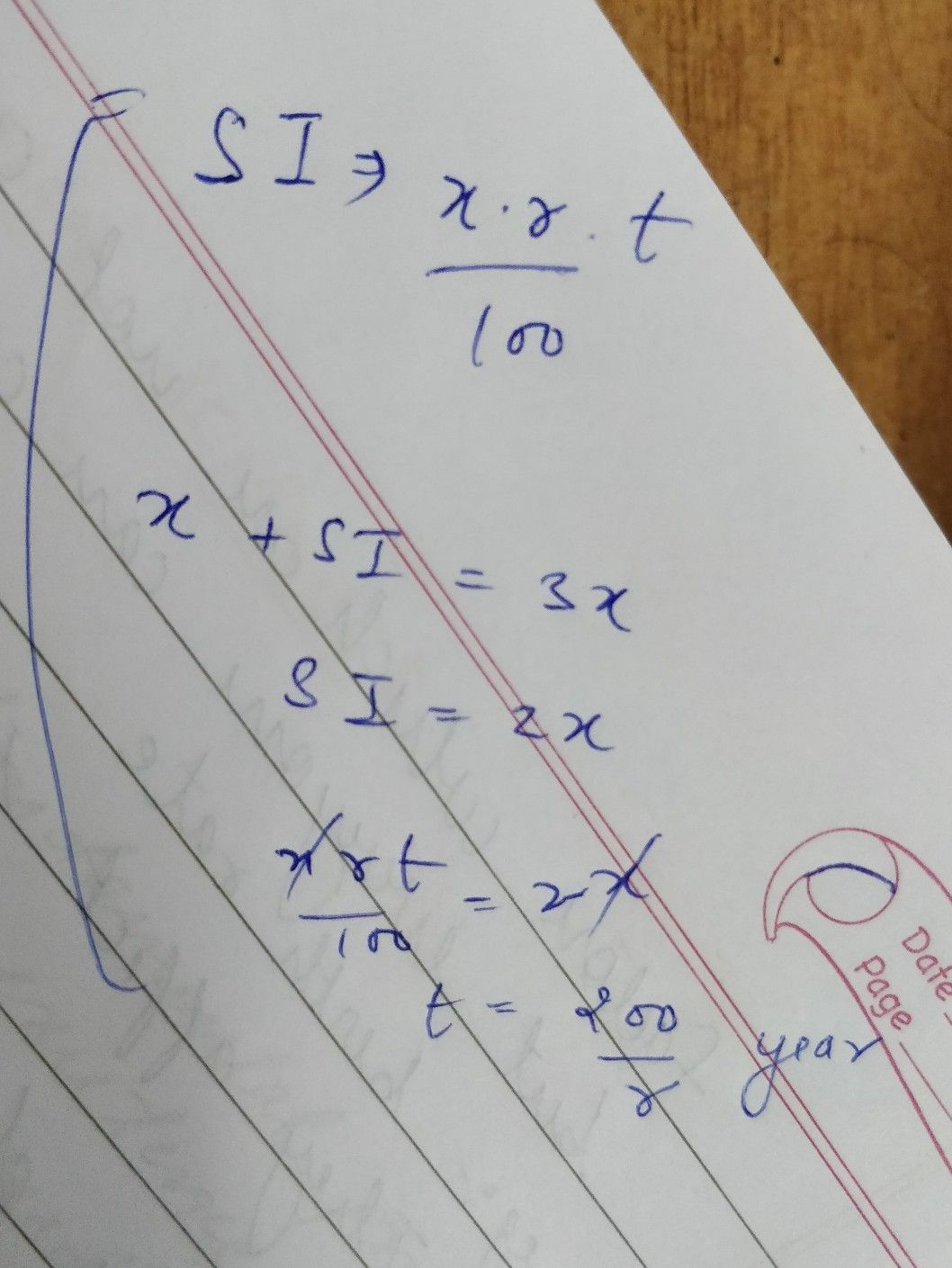Symbol
Problem$11$ $At$ what rate percent per annum will $a$ sum treble itself in $16$ years ? $12$ $At1$ what time will a sum of money put at simple interest treble itself? $13.$ If sum of money at simple interest doubles itself $in8$ years, find the rate p $14$ $A$ sum of money doubles itself in $6$ years. In how many years will it treble interest? $1^{5}$ What capital will earn a monthly interest of $q20$ at $6$ paise per rupee pe Divide 3 $6000$ into two parts so that the simple interest on the first part
10th-13th grade
Other
SolutionQanda teacher - Puru Gupta